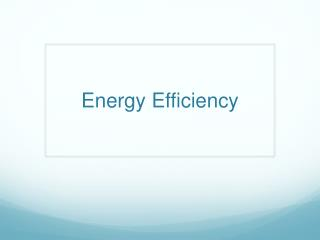DownloadDownload PresentationEnergy Efficiency

Energy Efficiency

Télécharger la présentationEnergy Efficiency

- - - - - - - - - - - - - - - - - - - - - - - - - - - E N D - - - - - - - - - - - - - - - - - - - - - - - - - - -
Presentation Transcript

1. Energy Efficiency

2. Energy Efficiency • the percentage of “wasted” energy to productive (output) energy in your isolated system (Inputs) (Outputs) (“Wasted” Energy)

3. Energy Efficiency Equation • How much energy is required to change the temperature • q=m(ΔT)c • q=amount of energy (measured in Joules) • m=mass (measured in grams) • ΔT= change in temperature (measured in degrees Celsius) • c= specific heat (measured in cal/g°C) Δ means “change in”

4. Specific Heat • Acertain number that tells us how much energy is required to raise 1 gram of a substance’s temperature 1 degree. • These numbers will always be the same for that substance. • Example: cwater= 1 cal/g°CORcwater=4.184 J/g°C • calories is the unit specific for heat energy (note: it is a lowercase c… this is important!) • We can still use Joules but c is a different number

5. Back to our equation… • q=m(ΔT)c • q=amount of energy (measured in Joules) • m=mass (measured in grams) • ΔT= change in temperature (measured in degrees Celsius) • c= specific heat (measured in cal/g°C) Example: How many calories of heat will need to be added to a 200g sample of water to raise its temperature 30°C? Step 1: Write down your givens: q= ? m= 200g ΔT= 30°C c=1 cal/g°C Step 2: Rewrite your equation: q=m(ΔT)c Step 3: Substitute your givens for all variables possible: q=(200)(30)(1) Step 4: Solve the algebra problem: q=6000 calories OR q=6 Calories (the large “C” is equal to 1000 “c”’s) Just like 1 Kilogram equals 1000 grams!

6. Example 2: Randy has a 500 g of water at 20°C. If he wants the final temperature of the water to be 75°C, how many Joules of heat will he need to add? Step 1: Write down your givens: q= ? m= 500g ΔT= 75°C-20°C = 55°C c=4.184 J/g°C Step 2: Rewrite your equation: q=m(ΔT)c Step 3: Substitute your givens for all variables possible: q=(500)(55)(4.184) Step 4: Solve the algebra problem: q= 115,060 Joules OR 115.06 Kilojoules To find the CHANGE in the temperature we subtract final temperature minus the initial temperature. We use 4.184 here because the problem asked for how many JOULES of heat we needed, not calories Just like 1 Kilogram equals 1000 grams!

7. Example 3: Harry has a 50g lump of unknown material. He experiments with the material and determines that the temperature of the substance increases by 6°C when 20 cal of heat are added. What is the substance’s specific heat? Step 1: Write down your givens: q= 20 cal m= 50g ΔT= 6°C c=? Step 2: Rewrite your equation: q=m(ΔT)c Step 3: Substitute your givens for all variables possible: 20=(50)(6)(c) Step 4: Solve the algebra problem: 20 = 300c 300 300 • 0.067 cal/g°C = c